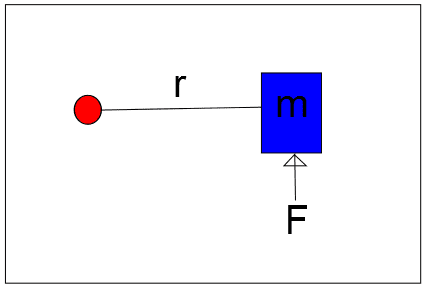# Jet on a rigid link question

• DrBwts

#### DrBwts

I have a mass (m) at the end of a rigid link of length r, pivoted at the red circle (below).

m is acted on by a force (F) which is always normal to the surface shown (thrust from a jet say).

My question is will this system reach a steady state, ie will it have constant angular velocity or will the angular acceleration just carry on increasing?

Thx NicHere's a thought: the gravitational field at the surface of the Earth is nearly constant - so if you drop a rock does it continue to gain speed as it falls?

Note that if air resistance is to be taken into account you there is a terminal velocity.

My question is will this system reach a steady state, ie will it have constant angular velocity or will the angular acceleration just carry on increasing?

Angular acceleration and angular velocity are different things. If we ignore air resistance and friction at the pivot, the angular acceleration will be constant and the angular velocity will keep on increasing without limit until something breaks.

If you consider air resistance, it is possible that the system will reach a steady state before something breaks. That force increases with speed, so as the speed increases will eventually be striping enough to counteract the applied force. (as UltrafastPED points out, this situation is analogous to the terminal velocity reached by a falling object in air).

OK thanks both. This is what I thought (sorry about the velocity/acceleration mix up).

Puzzling thing was, I ran this simulation in a well known CAD software & after an initial transitory increase in velocity it hit a steady state with no further acceleration!